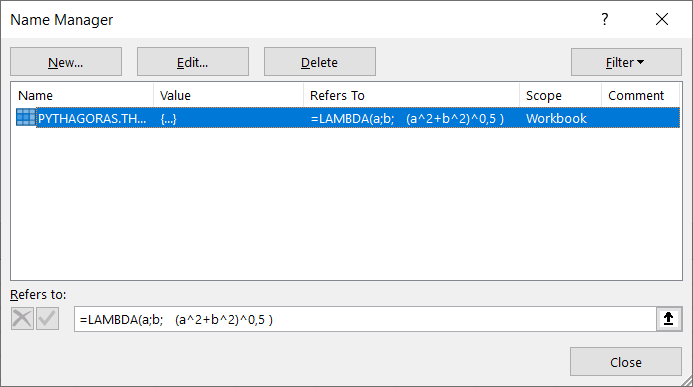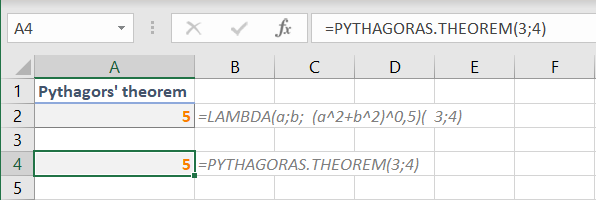# LAMBDA - create your own functions

To parameter, the outcreate a function calculating Pythagoras' theorem we need two inputs and one output.

The function LAMBDA first lists the input parameters and as the last, the output being the actual calculation.

=LAMBDA(a;b;(a^2+b^2)^0,5)

Appending another set of parenthesis acts as parameter input.

=LAMBDA(a;b;(a^2+b^2)^0,5)(3;4)

Using alt+Enter may make the formula easier to read.

=LAMBDA(a;b;

(a^2+b^2)^0,5

)(3;4)

Putting the lambda function into a defined name (Ctrl+3) makes it act like an internal function......and can be used as such with numbers as parameters or, as usual, using references to cells etc.Another example is finding the date for Midsummer.

=LAMBDA(year;

DATE(year;6;CHOOSE(WEEKDAY(DATE(year;6;1));21;20;26;25;24;23;22))

)(2022)

• LAMBDA

• CHOOSE
• WEEKDAY
• DATE